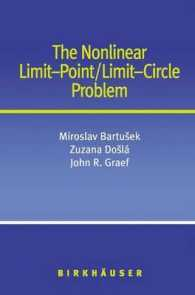### The Nonlinear Limit-Point/Limit-Circle Problem （2004. XII, 164 p.）

• 提携先の海外書籍取次会社に在庫がございます。通常3週間で発送いたします。
重要ご説明事項
1. 納期遅延や、ご入手不能となる場合が若干ございます。
2. 複数冊ご注文の場合、分割発送となる場合がございます。
3. 美品のご指定は承りかねます。
• 【重要：入荷遅延について】
ウクライナ情勢悪化・新型コロナウィルス感染拡大により、洋書・洋古書の入荷が不安定になっています。詳しくはこちらをご確認ください。
海外からのお取り寄せの場合、弊社サイト内で表示している標準的な納期よりもお届けまでに日数がかかる見込みでございます。
申し訳ございませんが、あらかじめご了承くださいますようお願い申し上げます。
• ◆画像の表紙や帯等は実物とは異なる場合があります。
• 製本 Paperback:紙装版/ペーパーバック版／ページ数 164 p.
• 商品コード 9780817635626

### Full Description

This self-contained monograph traces the evolution of the limit-point/limit-circle problem from its 1910 inception, in a paper by Hermann Weyl, to its modern-day extensions to the asymptotic analysis of nonlinear differential equations. The authors distill the classical theorems in the linear case and carefully map the progress from linear to nonlinear limit-point results. The relationship between the limit-point/limit-circle properties and the boundedness, oscillation, and convergence of solutions is explored, and in the final chapter, the connection between limit-point/limit-circle problems and spectral theory is examined in detail. With over 120 references, many open problems, and illustrative examples, this work will be valuable to graduate students and researchers in differential equations, functional analysis, operator theory, and related fields.

### Contents

1 Origins of the Limit-Point/Limit-Circle Problem.- 1.1 The Weyl Alternative.- 1.2 The Deficiency Index Problem.- 1.3 Second Order Linear Equations.- 2 Basic Definitions.- 2.1 Description of the Limit-Point/Limit-Circle Problem.- 2.2 Continuable and Singular Solutions.- 2.3 Extension of the LP/LC Properties to Singular Solutions.- 3 Second Order Nonlinear Equations.- 3.1 Introduction.- 3.2 The Superlinear Equation.- 3.2.1 Limit-Circle Criteria.- 3.2.2 Necessary Conditions for Limit-Circle behavior.- 3.2.3 Limit-Point Criteria.- 3.2.4 Necessary and Sufficient Conditions.- 3.2.5 The Superlinear Forced Equation.- 3.3 The Sublinear Equation.- 3.3.1 Limit-Circle Criteria.- 3.3.2 Limit-Point Criteria.- 3.3.3 Necessary and Sufficient Conditions.- 3.3.4 The Sublinear Forced Equation.- 3.4 Equations with r(t) ? 0.- 3.4.1 Nonlinear Limit-Point Results.- 3.4.2 Nonlinear Limit-Circle Results.- 4 Some Early Limit-Point and Limit-Circle Results.- 4.1 Wintner's Result.- 4.2 Early Results on Higher Order Linear Equations.- 4.2.1 Naimark's Results.- 4.2.2 Fedoryuk's Results.- 4.3 Nonlinear Limit-Point Results for Second Order Equations.- 4.4 Nonlinear Limit-Point Results for Higher Order Equations.- 4.5 Some New Generalizations of the Early Results.- 5 Relationship to Other Asymptotic Properties.- 5.1 Second Order Linear Equations.- 5.2 Second Order Nonlinear Equations.- 5.2.1 The Superlinear Case.- 5.2.2 The Sublinear Case.- 6 Third Order Differential Equations.- 6.1 Equations with Quasiderivatives.- 6.2 Linear Equations.- 6.3 Nonlinear Three-Term Equations.- 7 Fourth Order Differential Equations.- 7.1 Equations with Quasiderivatives.- 7.2 Sublinear Equations in Self-Adjoint Form.- 7.3 Two-Term Equations.- 7.4 Linear Equations.- 8 Nonlinear Differential Equations of n-th Order.- 8.1 Introduction.- 8.2 Basic Lemmas.- 8.3 Limit-Point Results.- 9 Relationship to Spectral Theory.- 9.1 Introduction.- 9.2 Self-Adjoint Linear Fourth Order Equations.- 9.3 Two-Term Even Order Linear Equations.- Author Index.